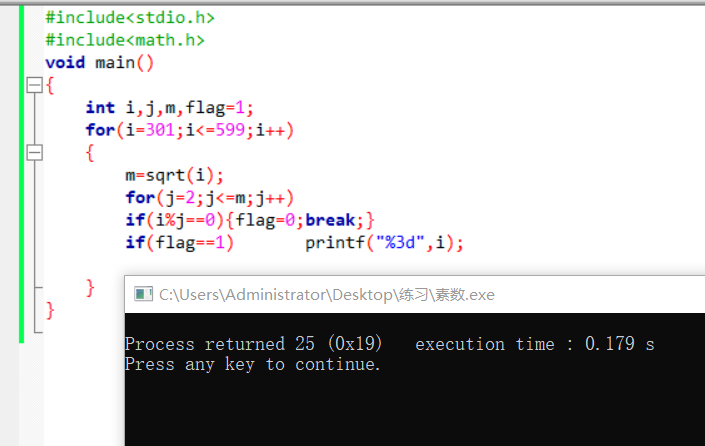2021-03-03 17:01

# c语言求301到599的素数为什么运行了怎么没结果• 点赞
• 写回答
• 关注问题
• 收藏
• 复制链接分享
• 邀请回答

#### 2条回答

• ``````#include<stdio.h>
#include<math.h>
void main()
{
int i,j,m,flag=1;
for(i=301;i<=599;i++)
{
m=sqrt(i);
for(j=2;j<=m;j++){
if(i%j==0){
flag=0;
break;
}
}
if(flag==1){
printf("%3d ",i);
}
flag=1;
}
}``````
点赞 评论 复制链接分享
• ``````#include<stdio.h>
#include<math.h>
void main()
{
int i,j,m,flag=1;
for(i=301;i<=599;i++)
{
m=sqrt(i);
for(j=2;j<=m;j++)
if(i%j==0){flag=0;break;}
if(flag==1)       printf("%3d",i);

}
}
``````
点赞 评论 复制链接分享